trustle > EngliSea > Corona Safe Schooling > mathematics

Math is Fun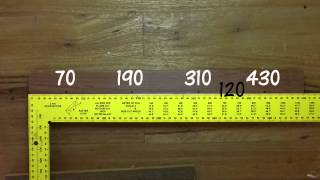addition tips and tricks 2
compensation and doubling
5048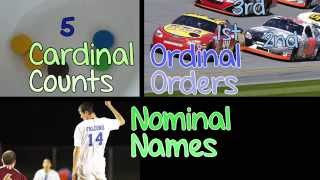arithmetic sequence
An arithmetic sequence is made by adding the same value each time. The value added each time is the common difference.
6266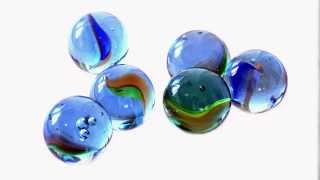cardinal, ordinal and nominal number
A cardinal number says how many of something there are. An ordinal number tells the position of something in a list. A nominal number is a number used like a name.
8948dimension
The number of dimensions is how many values are needed to locate points on a shape.
5044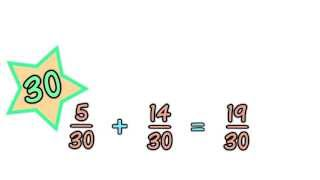least common denominator
The denominator is the bottom number in a fraction. It shows how many equal parts the item is divided into.
8951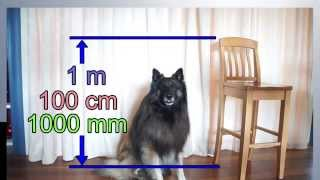metric length
We can measure how long things are, or how tall or how far apart they are. The meter is the basic measurement of length in the metric system. How far is it across this patch of grass?
5046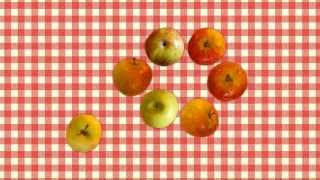Prime and Composite Number
○ It is a prime number when it can't be divided evenly by any number except 1 or itself. ○ prime, composite, whole, factor, fraction
8949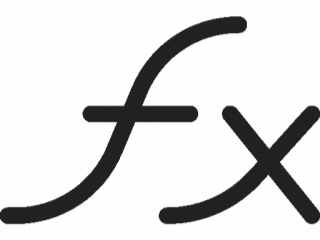Quick Math Index
arithmetic, geometry, algebra, exponentiation, logarithm, trigonometry, calculus, topology
5043#4758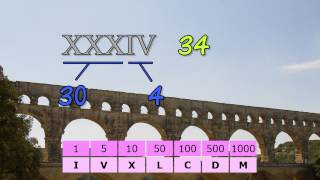Roman numeral
The Romans showed numbers into the thousands using these 7 symbols: I for 1, V for 5, X for 10, L for 50, C for 100, D for 500 and M for 1000.
5045triangles
Equilateral, Isosceles and Scalene
3961-